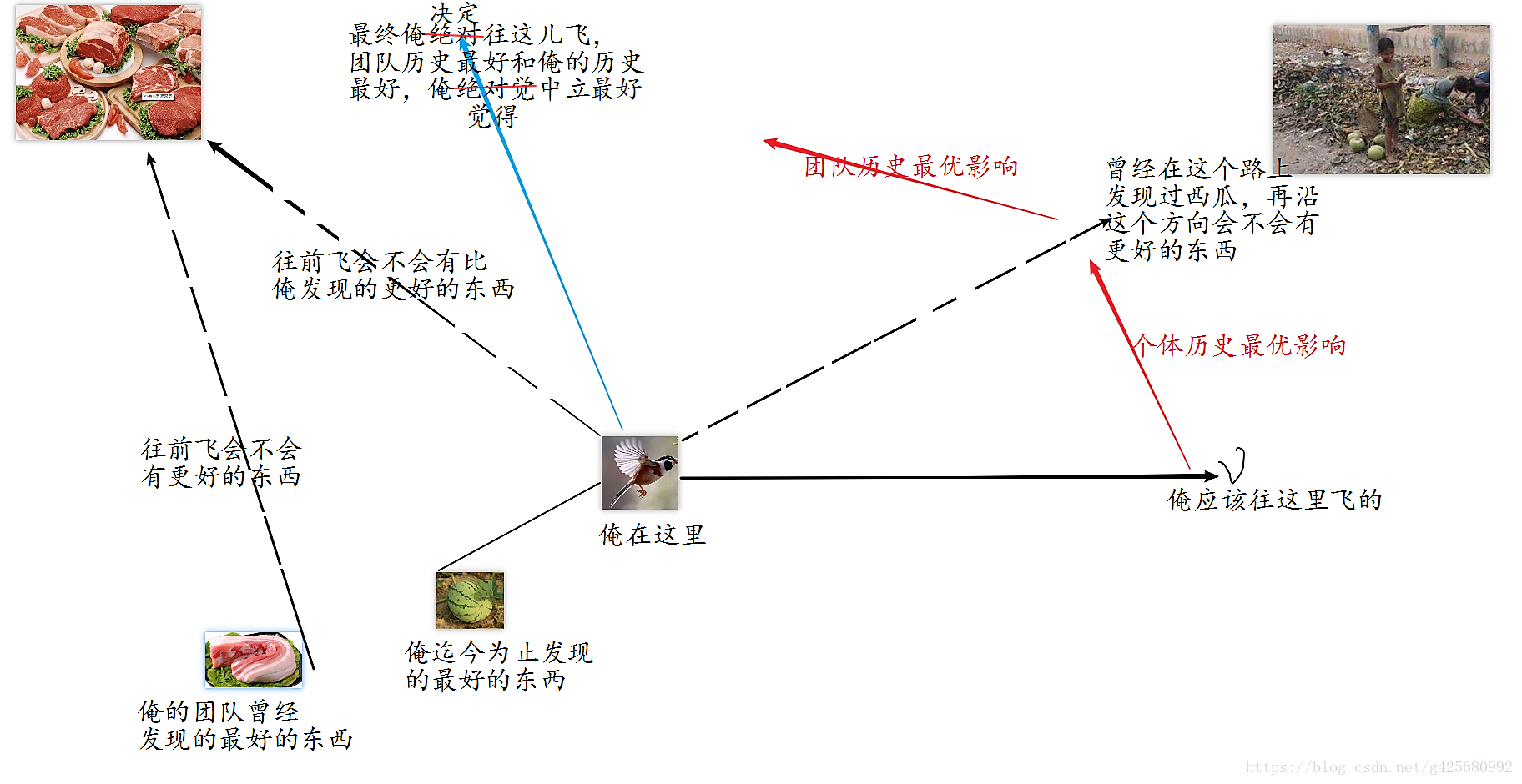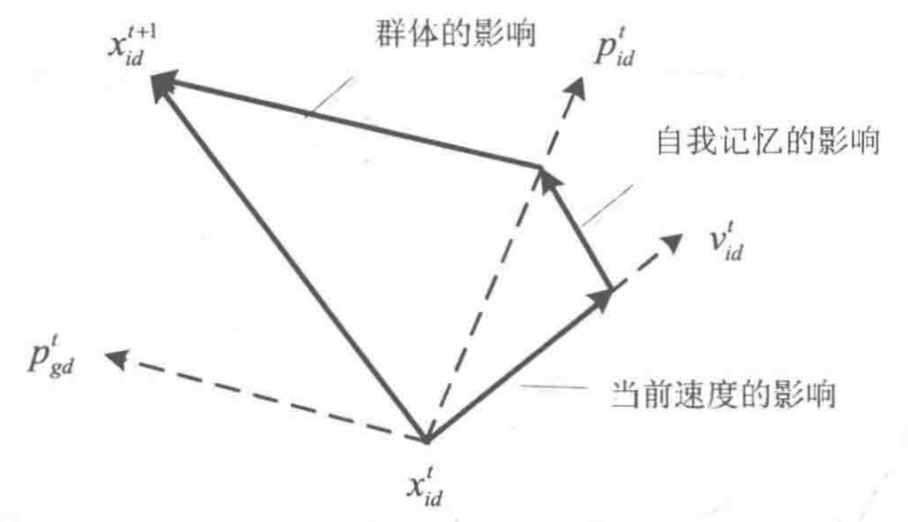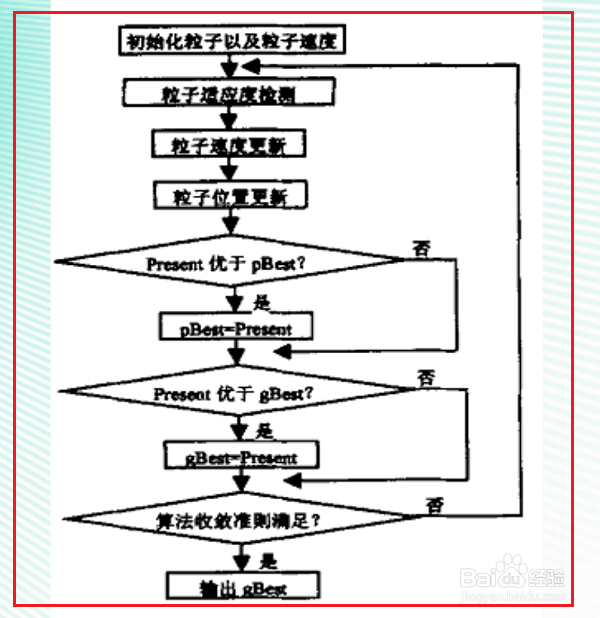1 篇文章 0 订阅

# 经典智能算法之粒子群算法

## 基本思想

POS 初始化为一群随机粒子(随机解)。然后通过迭代找到最优解在每一次迭代中，粒子通过跟踪两个"极值"来更新自己。第一个就是粒子本身所找到的最优解，这个解叫做个体极值。另一个极值是整个种群目前找到的最优解，这个极值是全局极值。另外也可以不用整个种群而只是用其中一部分作为粒子的邻居，那么在所有邻居中的极值就是局部极值。

## 迭代公式

v i d = w ∗ v i d + c 1 r 1 ( p i d − x i d ) + c 2 r 2 ( p g d − x i d ) v_{id} = w*v_{id} + c_1r_1(p_{id}-x_{id})+c_2r_2(p_{gd}-x_{id})
x i d = x i d + v i d x_{id} = x_{id} + v_{id}

### （1）大体了解一下

1. 第一部分代表了粒子保持先前速度的趋势，相当于物理学的惯性或者动量

2. 第二部分代表了粒子对自身经验的记忆，相当于生物学意义上的认知

3. 第三部分代表了粒子之间协同的社会影响，相当于群体学上的社会

x t + 1 = x t + v t x_{t+1}=x_t+v_t

### （2）认识各个参数

——w惯性权重

——c1，c2学习因子

——r2，r2 [0,1]的随机数

（上述参数是怎么设置，又该怎么算，我一窍不通哈，可以忽略不看）

——Pid，Pgd 两极值就是前面说的两个极值，千万别弄错了

#### 2. 这个极值怎么表示

……

X i = ( x i 1 , x i 2 , . . . , x i D ) X_i=(x_{i1},x_{i2},...,x_{iD})

### （3）通俗理解算法## 程序设计

### 初始化

for i=1:N
for j=1:D
x(i,j) = randn;   %随机初始化位置
v(i,j) = randn;   %随机初始化速度
end
end



### 找极值

#### 全局极值

for i=1:N
p(i) = fitness(x(i,:)); %x(i,:)即代表单个的粒子哦
y(i,:) = x(i,:);
end

pg=x(N,:);               %全局最优
for i = i:(N-1)
if fitness(x(i,:)) < fitness(pg)
pg = x(i,:);
end
end



#### 个体极值

for t = 1:M
for i = 1:N
v(i,:) = w*v(i,:) + c1*rand*(y(i,:)-x(i,:)) + c2*rand*(pg - x(i,:));
x(i,:) = x(i,:) + v(i,:);
if fitness(x(i,:)) < p(i)
pi = fitness(x(i,:));
y(i,:) = x(i,:);
end
if p(i) < fitness(pg)
pg = y(i,:);
end
end
Pbest(t) = fitness(pg);
endCSDN博客

#### 替精神支柱大猫仔化缘了，喵~04-21
06-0428万+06-161292
09-191376
11-061万+
01-281001
08-0327万+
02-073704
09-09
12-029万+
06-11
05-262604
01-109018
11-242103
02-152万+
09-122674
06-277万+
01-194887点击重新获取扫码支付余额充值